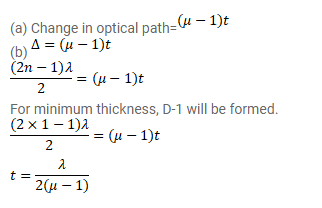# A plate of thickness t made of a material of refractive

Question:

A plate of thickness $t$ made of a material of refractive index $\mu$ is placed in front of one of the slits in a double slit experiment. (a) Find the change in the optical path due to introduction of the plate. (b) What should be the minimum thickness $t$ which will make the intensity at the centre of the fringe pattern zero? Wavelength of the light used is $\lambda$. Neglect any absorption of light in the plate.

Solution: